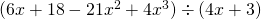Chapter 6: Polynomials

# 6.7 Dividing Polynomials

Dividing polynomials is a process very similar to long division of whole numbers. But before looking at that, first master dividing a polynomial by a monomial. The way to do this is very similar to distributing, but the operation to distribute is the division, dividing each term by the monomial and reducing the resulting expression. This is shown in the following examples.

Example 6.7.1

Divide the following:

1.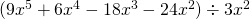Breaking this up into fractions, we get: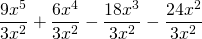Which yields: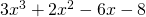2.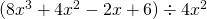Breaking this up into fractions, we get: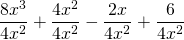Which yields: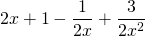Long division is required when dividing by more than just a monomial. Long division with polynomials is similar to long division with whole numbers.

Example 6.7.2

Divide the polynomial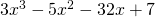by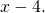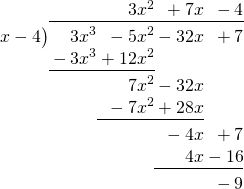The steps to get this result are as follows:

1. Divide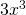by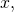yielding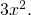Multiply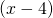by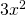, yielding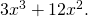Subtract and bring down the next term and repeat.
2. Divide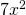byyielding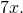Multiplyby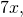yielding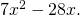Subtract and bring down the next term and repeat.
3. Divide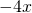byyielding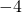. Multiplyby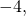yielding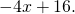Subtract.

The solution can be written as either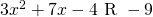or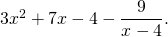The more formal way of writing this answer is the second option.

Example 6.7.3

Divide the polynomial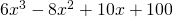by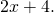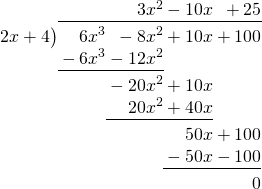The steps to get this result are as follows:

1. Divide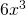by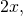yieldingMultiply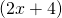by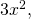yielding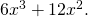Subtract and bring down the next term and repeat.
2. Divide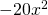byyielding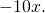Multiplyby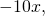yielding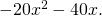Subtract and bring down the next term and repeat.
3. Divide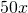byyielding 25. Multiplyby 25, yielding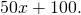Subtract.

The solution is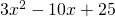with no remainder.

# Questions

Solve the following polynomial divisions.

1.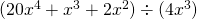2.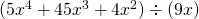3.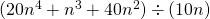4.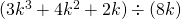5.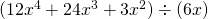6.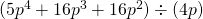7.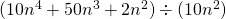8.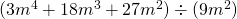9.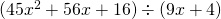10.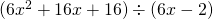11.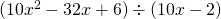12.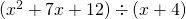13.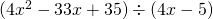14.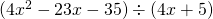15.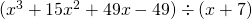16.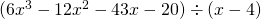17.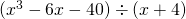18.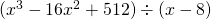19.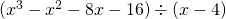20.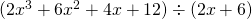21.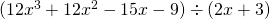22.